## php 如何更新数组，并限制十一个元素

xuweijia90324 发布于 2016/05/26 14:58

更新的数组：array('cat'=>'blue','dog'=>'yellow','a'=>'aaa','b'=>'bbb')

1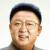```<?php

\$input = ['a' => 'aaa', 'b' => 'bbb', 'c' => 'ccc', 'd' => 'ddd',];
\$add = ['cat'=>'blue','dog'=>'yellow',];
\$output = array_merge(\$add, array_slice(\$input, 0, -2));
var_dump(\$output);```

0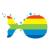```\$a = array('a'=>'aaa','b'=>'bbb','c'=>'ccc','d'=>'ddd');
\$b = array('cat'=>'blue','dog'=>'yellow',4,3,65,5,40,11,2,66,15,34,235,667);
\$a_count = count(\$a);
\$b_count = count(\$b);
if(\$b_count > 11){
\$b = array_slice(\$b, 0, 10);
}
if(\$a_count > 11){
\$a  = array_slice(\$a, 0, 10);
}
\$new_arr = array();
foreach (\$b as \$k => \$v) {
\$new_arr[\$k] = \$v;
}
for (\$i = 0; \$i < count(\$b); \$i++) {
array_pop(\$a);
}
foreach (\$a as \$k => \$v) {
\$new_arr[\$k] = \$v;
}

\$a = \$new_arr;
var_dump(\$a);```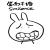b 数组前几个没有更新，后面的倒是踢出了，不符合要求啊
0```\$a = array('a'=>'aaa','b'=>'bbb','c'=>'ccc','d'=>'ddd');
\$b = array('cat'=>'blue','dog'=>'yellow',4,3,65,5,40,11,2,66,15,34,235,667);
\$new_arr = array();
foreach (\$a as \$k => \$v) {
\$new_arr[\$k] = \$v;
}
foreach (\$b as \$k => \$v) {
\$new_arr[\$k] = \$v;
}
\$new_arr = array_slice(\$new_arr, 0, 11);
var_dump(\$new_arr);```
0```或是
\$a = array('a'=>'aaa','b'=>'bbb','c'=>'ccc','d'=>'ddd');
\$b = array('cat'=>'blue','dog'=>'yellow',4,3,65,5,40,11,2,66,15,34,235,667);
\$new_arr = array_merge(\$a,\$b);
\$new_arr = array_slice(\$new_arr, 0, 11);
var_dump(\$new_arr);```

0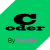http://php.net/manual/zh/class.splfixedarray.php

0```<?php
class MyArray extends SplFixedArray{
public function push(\$value){
for(\$i = \$this->getSize()-1; \$i >=1; \$i--){
\$this[\$i] = \$this[\$i-1];
}
\$this = \$value;
}
}

\$arr = new MyArray(11);

for(\$i = 0; \$i < 11; \$i++){
\$arr->push(\$i);
}

var_dump(\$arr);

// object(MyArray)#1 (11) { => int(10) => int(9) => int(8) => int(7) => int(6) => int(5) => int(4) => int(3) => int(2) => int(1) => int(0) }

\$arr->push('我就是来测试的');

var_dump(\$arr);

// object(MyArray)#1 (11) { => string(21) "我就是来测试的" => int(10) => int(9) => int(8) => int(7) => int(6) => int(5) => int(4) => int(3) => int(2) => int(1) }```

0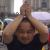0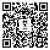#### 引用来自“inuxor”的评论

```<?php

\$input = ['a' => 'aaa', 'b' => 'bbb', 'c' => 'ccc', 'd' => 'ddd',];
\$add = ['cat'=>'blue','dog'=>'yellow',];
\$output = array_merge(\$add, array_slice(\$input, 0, -2));
var_dump(\$output);```